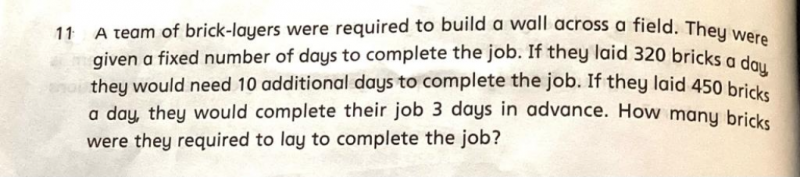# Question1 day 320 bricks   need another 10 days             => Bricks  to lay further  = 320 x 10 = 3200

1 day 450 bricks    complete 3 days in advance => Bricks no need to lay = 450 x 3 = 1350

Total excess/shortage bricks to be laid = 3200 + 1350  = 4550

Difference in the bricks = 450 – 320 = 130

Fixed days to complete the job = 4550 / 130 = 35

Completed 3 days in advance => 35 – 3 = 32

Therefore, total bricks = 450 x 32 = 14,400

Check:

35 x 320 = 11,200

14400-11200 = 3200  => 10 additional days required.